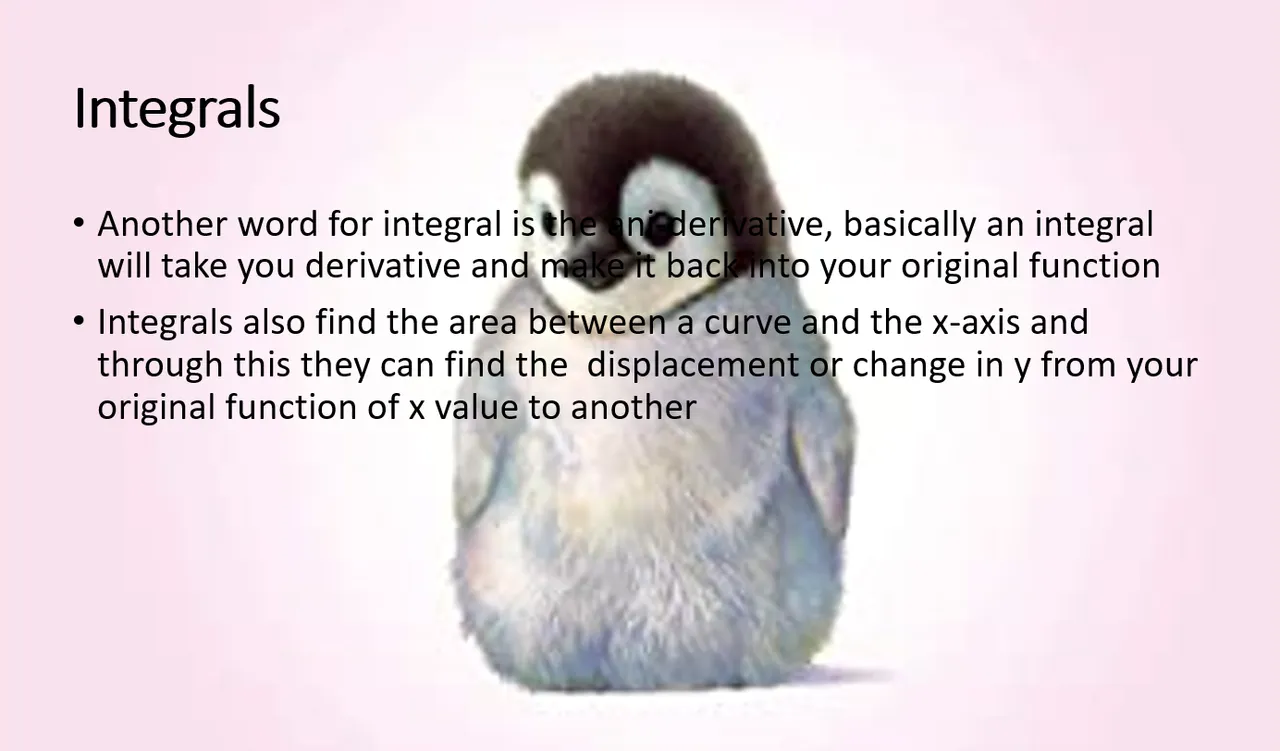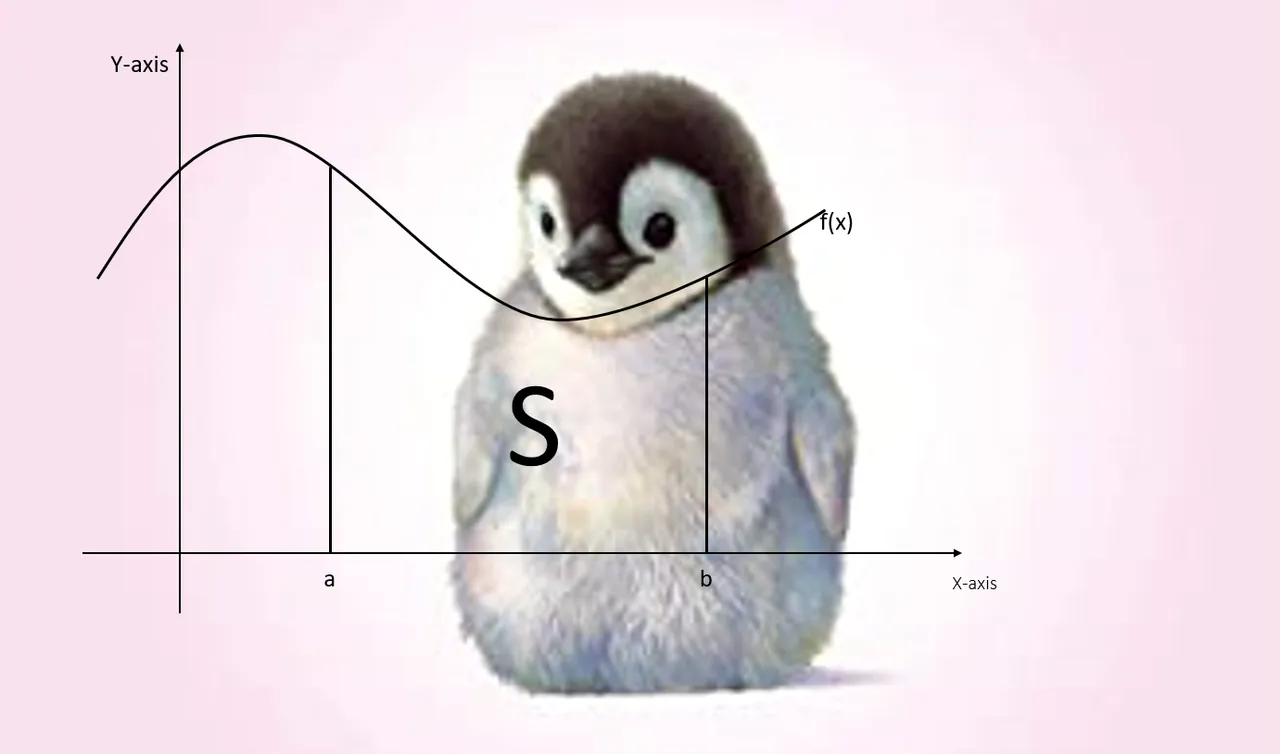# Integrals

Acquaintance with the "simple" integral. What are integrals? and
what are they used for?S is the region that lies under the graph y=f(x) from a to b. This means that S is bounded by the graph of a continuous function f, the vertical lines x=a to x=b ad the x-axis.H2
H3
H4
3 columns
2 columns
1 column
Join the conversion now